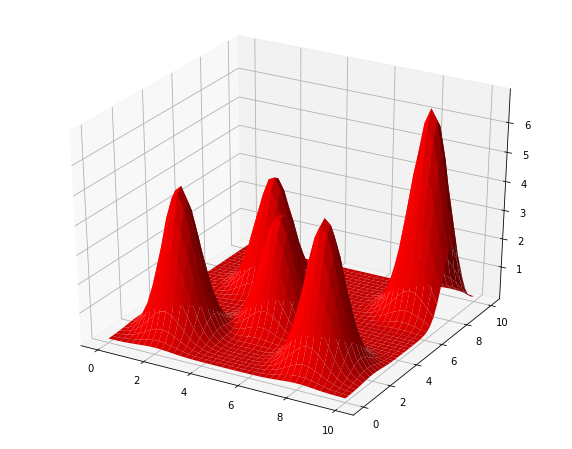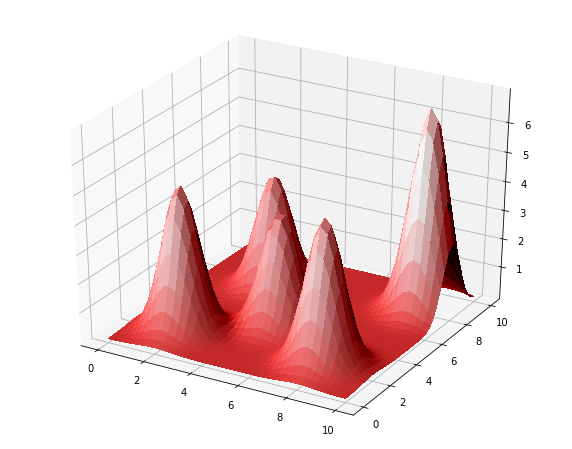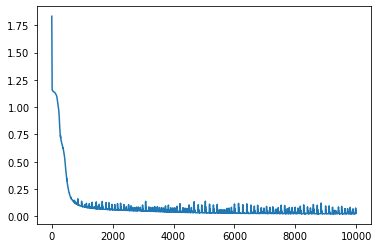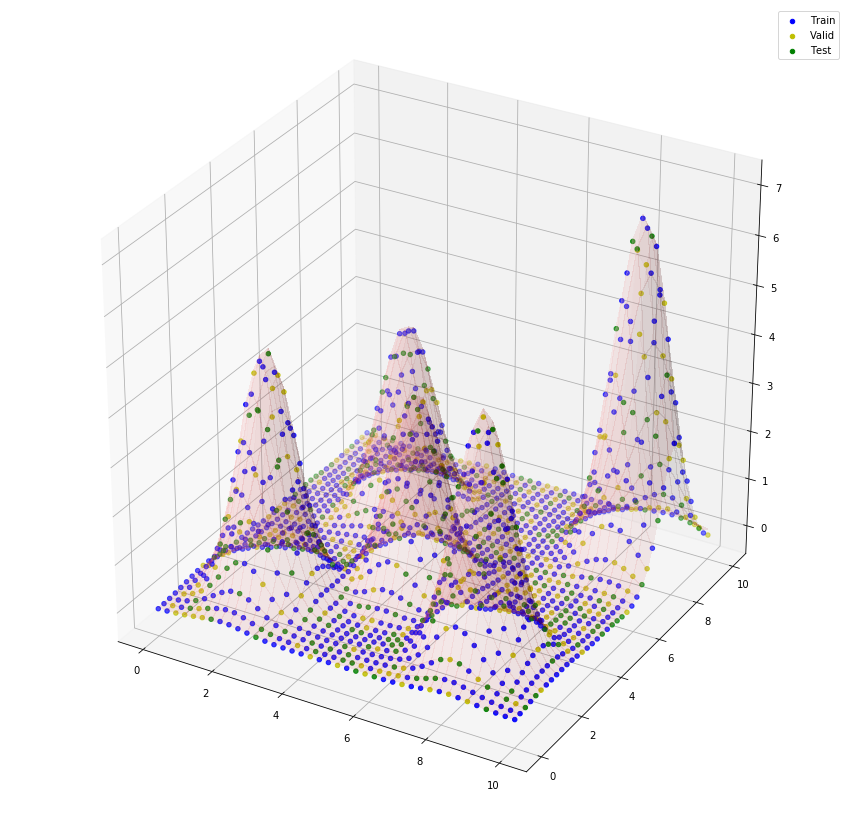# Optimizers, Data Partitioning, Finding Good Parameters¶

07.2:

• Changes indexing used to create final fold.
In :
import numpy as np
import matplotlib.pyplot as plt


We have discussed how we can create a vector of weight values and views onto parts of the vector to define the weight matrices for each layer of a neural network. Here is a function that will do that, given a list of shapes of the matrices of weights for each layer.

In :
def make_weights_and_views(shapes):
# vector of all weights built by horizontally stacking flatenned matrices
# for each layer initialized with uniformly-distributed values.
all_weights = np.hstack([np.random.uniform(size=shape).flat / np.sqrt(shape)
for shape in shapes])
# Build list of views by reshaping corresponding elements from vector of all weights
# into correct shape for each layer.
views = []
start = 0
for shape in shapes:
size =shape * shape
views.append(all_weights[start:start + size].reshape(shape))
start += size
return all_weights, views


What would the shapes of the weight matrices be of a neural network with 2 inputs, 2 hidden layers with 20 and 10 units, respectively, and 1 output?

In [ ]:


In [ ]:


In [ ]:



Now let's make some data with 2 inputs per sample and 1 target output. We can have fun with this by making target values as the height of several hills over the 2-dimensional base plane of input values.

In :
centers = np.array([[2,2], [5,4], [8,2], [9,8], [3,7]])
heights = np.array([5, 4, 5, 7, 4])

In :
def calc_heights(X, center):
diffv = X - center
return np.exp(- np.sum(diffv * diffv, axis=1)).reshape(-1, 1)

In :
X_1 = np.linspace(0,10, 20)
X_2 = np.linspace(0,10, 20)
X_1_mesh, X_2_mesh = np.meshgrid(X_1, X_2)
print(X_1_mesh.shape, X_2_mesh.shape)
X = np.hstack((X_1_mesh.reshape((-1, 1)), X_2_mesh.reshape((-1, 1))))
print(X.shape)

(20, 20) (20, 20)
(400, 2)

In :
h = calc_heights(X, centers)
h.shape

Out:
(400, 1)
In :
from mpl_toolkits.mplot3d import Axes3D
from matplotlib import cm
from matplotlib.ticker import LinearLocator, FormatStrFormatter

fig = plt.figure(figsize=(10, 8))

n = 40
X_1 = np.linspace(0, 10, n)
X_2 = np.linspace(0, 10, n)
X_1_mesh, X_2_mesh = np.meshgrid(X_1, X_2)
X = np.hstack((X_1_mesh.reshape((-1, 1)), X_2_mesh.reshape((-1, 1))))
Z = np.zeros((X.shape, 1))
for hilli in range(len(heights)):
Z += calc_heights(X, centers[hilli]) * heights[hilli]
Z_mesh = Z.reshape(n, n)

surf = ax.plot_surface(X_1_mesh, X_2_mesh, Z_mesh, rstride=1, cstride=1, color='red', linewidth=0);Or, how about some more realistic lighting? See these examples of using LightSource.

In :
from matplotlib.colors import LightSource
# LightSource?

In :
fig = plt.figure(figsize=(10, 8))

white = np.ones((Z_mesh.shape, Z_mesh.shape, 3))
red = white * np.array([1, 0, 0])
green = white * np.array([0, 1, 0])
blue = white * np.array([0, 0, 1])

ls = LightSource(azdeg=-20, altdeg=70)

rgb = ls.shade_rgb(red, Z_mesh, vert_exag=0.1) #, blend_mode='soft')

surf = ax.plot_surface(X_1_mesh, X_2_mesh, Z_mesh, rstride=1, cstride=1, facecolors=rgb,Now we can make some data. X is our base plane points, which will be the inputs. The target values for T are the heights.

In :
T = Z
X.shape, T.shape

Out:
((1600, 2), (1600, 1))

Before trying to fit this data with our neural network model, let's discuss how to partition data into training, validation and testing. Steps involved are:

1. shuffle the samples into a random order,
2. partition the data into $n$ folds,
3. assign the first fold to the validation set,
4. assign the second fold to the testing set, and
5. collect the third through the last folds into a training set.
In :
rows = np.arange(X.shape)
np.random.shuffle(rows)  # shuffle the row indices in-place (rows is changed)
X = X[rows, :]
T = T[rows, :]

n_folds = 5
n_samples = X.shape
n_per_fold = n_samples // n_folds
n_last_fold = n_samples - n_per_fold * (n_folds - 1)  # handles case when n_samples not evenly divided by n_folds

folds = []
start = 0
for foldi in range(n_folds-1):
folds.append( (X[start:start + n_per_fold, :], T[start:start + n_per_fold, :]) )
start += n_per_fold
folds.append( (X[start:, :], T[start:, :]) )   # Changed in notes 07.2
len(folds), len(folds), folds.shape, folds.shape

Out:
(5, 2, (320, 2), (320, 1))
In :
Xvalidate, Tvalidate = folds
Xtest, Ttest = folds
Xtrain, Ttrain = np.vstack([X for (X, _) in folds[2:]]), np.vstack([T for (_, T) in folds[2:]])
Xtrain.shape, Ttrain.shape, Xvalidate.shape, Tvalidate.shape, Xtest.shape, Ttest.shape

Out:
((960, 2), (960, 1), (320, 2), (320, 1), (320, 2), (320, 1))

Once you have completed the NeuralNetwork class definition in assignment A2, you can use it like this. Assume you have saved your NeuralNetwork class in a file named neuralnetwork.py (not required for the assignment).

In :
import neuralnetwork as nn

In :
nnet = nn.NeuralNetwork(X.shape, [10, 5], T.shape)
nnet

Out:
NeuralNetwork(2, [10, 5], 1)
In :
nnet.train(Xtrain, Ttrain, n_epochs=10000, learning_rate=0.01, method='adam')

Adam: Epoch 1000 Error=0.26271

Out:
NeuralNetwork(2, [10, 5], 1)
In :
import time

startTime = time.time()

nnet = nn.NeuralNetwork(Xtrain.shape, [20, 10, 5], Ttrain.shape)

print('Training took {:.2f} seconds.'.format(time.time()-startTime))

plt.plot(nnet.error_trace);

Adam: Epoch 1000 Error=0.09674
Training took 14.40 seconds.In :
def rmse(A, B):
return np.sqrt(np.mean((A - B)**2))

In :
print(f'RMSE Training {rmse(Ttrain, nnet.use(Xtrain)):.3f}')
print(f'RMSE Validation {rmse(Tvalidate, nnet.use(Xvalidate)):.3f}')
print(f'RMSE Testing {rmse(Ttest, nnet.use(Xtest)):.3f}')

RMSE Training 0.035
RMSE Validation 0.036
RMSE Testing 0.040

In :
fig = plt.figure(figsize=(15, 15))

white = np.ones((Z_mesh.shape, Z_mesh.shape, 3))
red = white * np.array([1, 0, 0])
green = white * np.array([0, 1, 0])
blue = white * np.array([0, 0, 1])

ls = LightSource(azdeg=-20, altdeg=70)

rgb = ls.shade_rgb(red, Z_mesh, vert_exag=0.1) #, blend_mode='soft')

surf = ax.plot_surface(X_1_mesh, X_2_mesh, Z_mesh, rstride=1, cstride=1, facecolors=rgb,
linewidth=0, antialiased=False, shade=True, alpha=0.1)  # alpha < 1 is transparent

Ytrain = nnet.use(Xtrain)
ax.scatter(Xtrain[:, 0], Xtrain[:, 1], Ytrain, c='b', marker='o', label='Train')

Yvalidate = nnet.use(Xvalidate)
ax.scatter(Xvalidate[:, 0], Xvalidate[:, 1], Yvalidate, c='y', marker='o', label='Valid')

Ytest = nnet.use(Xtest)
ax.scatter(Xtest[:, 0], Xtest[:, 1], Ytest, c='g', marker='o', label='Test')

plt.legend();Can we visualize what the hidden units have learned, like we did for data sets with a single variable input?

Sure. Just plot a unit's output as a surface over the base plane of input values.

In :
plt.figure(figsize=(25, 25))

nPlot = 40
Xplot = np.linspace(0, 10, nPlot)
Yplot = np.linspace(0, 10, nPlot)
Xplot, Yplot = np.meshgrid(Xplot, Yplot)
XYplot = np.hstack((Xplot.reshape((-1, 1)), Yplot.reshape((-1, 1))))
white = np.ones((Xplot.shape, Xplot.shape, 3))
red = white * np.array([1, 0, 0])

Ys = nnet.forward_pass(XYplot)
Ys = Ys[1:]  # to remove inputs

nUnits = sum(nnet.n_hiddens_per_layer)
nPlotsSqroot = int(np.sqrt(nUnits))
if nPlotsSqroot**2 <= nUnits:
nPlotsSqroot += 1

ls = LightSource(azdeg=-20, altdeg=70)

# ls = LightSource(azdeg=90, altdeg=80)
white = np.ones((Xplot.shape, Xplot.shape, 3))
red = white * np.array([1, 0, 0])

fig = plt.figure(figsize=(10, 10))
ploti = 0
surfaces = []
for layeri in range(len(nnet.n_hiddens_per_layer)):
for uniti in range(nnet.n_hiddens_per_layer[layeri]):
ploti += 1
ax = fig.add_subplot(nPlotsSqroot, nPlotsSqroot, ploti, projection='3d')
Yunit = Ys[layeri][:, uniti].reshape(Xplot.shape)

<Figure size 1800x1800 with 0 Axes>